# Decision making statements and Loops in C Programming

A normal program is not a sequential execution of expressions or statements one after the other. It will have certain conditions to be checked or it will have certain number of iterations. When we check for certain conditions to execute further then it called as decision statements. If the condition in decision statements are true then one set of instructions are executed, if false then another set of instructions are executed. This kind of decision is cannot be done using conditional operator. That will be mainly used to return single line of action / result. When we have to perform series of operation, we use IF conditions.

When we have to execute particular set of instructions multiple times or known number of times (iterations), then we use FOR loops or DO/WHILE loops.

Details about all these are discussed below:

## If… Else Statements

These are the decision making statements.  It is used for checking certain condition to decide which block of code to be executed. The general syntax for if..else statement is as follows:

```if (condition / s){
expressions / statements;
} else {
expressions / statements;
}```
```if (condition / s){
expressions / statements;
} else if {
expressions / statements;
} else {
expressions / statements;
}```

The flow of code for If condition is as shown below. It verifies the condition first. If it returns TRUE value, then it continues to execute the set of expressions/statements within it, else it jumps to execute other set of expressions/statements.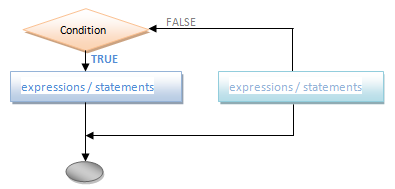If statement can take different forms. We can have single if condition, without else part. This is used when we have only set of statements to be executed when the condition is true and there is no statement for false conditions. i.e.;

```if (condition / s){
expressions / statements;
}```

Eg :

```if (intDivisor == 0) {
printf ("Warning!: Divisor is Zero!!\n Please re-enter the divisor :");
scanf ("%d", &intDivisor);
}```

intResult=intDividend / intDivisor;

In above case it checks for the divisor and warns if it is zero and asks for re-entering the number. Otherwise it proceeds with normal execution of program by dividing the numbers.

If statement can have expressions to be executed when the condition is false. Those expressions are added in the else part of the ‘if’ statement.

```if (condition / s) {
expressions / statements;
} else {
expressions / statements;
}```

eg :

```if (intVal >= 0)
printf ("You entered a Positive Number!");
else
printf ("You entered a Negative Number!");```

Here it checks for the number is positive or negative and displays the message accordingly. Here we can note that we have not used curly brackets. This is because we have only one statement to be executed within if and else statements. If we have more than one expression / statements to be executed, then we need to include within curly brackets. These curly braces indicate that they are one set of expression, which needs to be executed as part of condition.

We can have if statement within another if statement, i.e.; we can have nested if statements.
e.g.: –

```if (intVal >= 0)
if (intVal == 0)
printf ("You entered a Zero!");
else
printf ("You entered a Positive Number!");
else
printf ("You entered a Negative Number!");```

Here it first checks for the positive number and then within it, again checks for the zero and non-zero numbers. This kind of nesting need not be in if part alone, but can also be on else part too.

```if (intVal < 0)
printf ("You entered a Negative Number!");
else
if (intVal == 0)
printf ("You entered a Zero!");
else
printf ("You entered a Positive Number!");```

This kind if nesting may look little complicated. We can make this little better by combining the else and nested if together like below :

```if (intVal < 0)
printf ("You entered a Negative Number!");
else if (intVal == 0)
printf ("You entered a Zero!");
else
printf ("You entered a Positive Number!");```

Now this gives better understanding of the code. We can write the code in any of the above methods, depending on the requirement.

## For Loops

Suppose we have to enter name a student into the program. What will we do is, we will write a message to enter the name and enter the name from keyboard while executing. Suppose we have to enter 15 such student names. What will we do now? Will we be writing scanf for 15 times? Imagine if the number of students is even more? Can we go writing scanf for so many times? What if we miss the count in between? This will result in wrong result as well as confusion to the developer / user. It simply increases the code length too.

Here the process / task are same for all the 15 or more number of students. We have to enter the names 15 times from the keyboard. The command to do this is scanf, irrespective of the number of times. That means, it’s a repetition of executing the statement scanf. Hence we can have some loop, which executes this scanf for the known number of times – here 15 or more depending on the number of students to be entered.

This kind of iteration to execute same set of expression/ statements is done by using FOR loops. This for loop can be used when we know the number of iterations or till it satisfies certain conditions. The general syntax for ‘For’ loop is given below :

```for (intial_value; condition; increment_factor) {
statement/s;
}```

Here, initial_value sets the initial value for the iteration. It can be declared and initialized within for loop itself – that is while we are setting initial value inside the ‘for’ loop. This is the initial step to be executed within for loop and is executed only once. It indicates from where the execution should begin. This can be left blank, if it is initialized outside the loop or no need to have any initial value. It is then followed by semicolon to indicate the end of initial value.

Next is the conditional check for the iteration. This is responsible for checking the condition and iterating the statements within the ‘for’ loop – body of loop. If the condition is TRUE, then it executes the steps within it. If the condition fails or return FALSE, then it exits the loop and proceeds with next set of statements outside the loop. There can be one or more conditions here, joined together by using logical operators. Conditions can be any expression or statement using any of the operators.  This condition is repeatedly checked for each iterations of the loop. This is followed by semicolon to indicate the end of it.

Next is the increment factor which is used to increment the loop counter. This increases/decreases the iterations in the loop.

The flow chart for the execution of the for loop is shown below :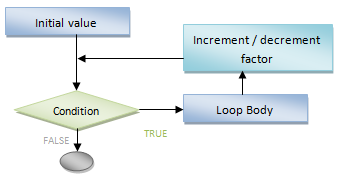Let us consider an example to understand how for loops work. Consider a program to display first 15 natural numbers on the screen.

```#include

void main () {
int intNum;

printf ("\n First 15 natural numbers are: \n");
for (intNum = 0; intNum < 15; intNum++) {
printf ("%d  ", intNum);
}
}```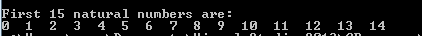In the above program, for loop initializes the value for intNum as intNum = 0. When the loop begins, it considers this initial value and checks for the condition – intNum<15, which returns TRUE. Hence executes the printf statement within it for intNum = 0. Once it is done, it increments the value of intNum by 1. Now it does not consider intNum = 0 anymore. This value is considered only once at the beginning. It checks for the n=incremented value is less than 15 or not. If yes, it continues to print the value and increments intNum. This process will go on till intNum = 15. When intNum = 15, it checks for the condition intNum

Suppose we have initialized intNum while declaring itself. Then no need to re-initialize its value in the for loop unless and until its initial value for the loop is different. (there is no harm in re-initializing). Then above program can be re-written as below :

```#include

void main () {
int intNum = 0;

printf ("\n First 15 natural numbers are: \n");
for ( ; intNum < 15; intNum++) {
printf ("%d  ", intNum);
}
}```Note here we have left blank for intial_value followed by semicolon to indicate that there is no initial value and the next one is the condition for the loop.

Suppose we have not declared intNum at the beginning. We can declare and initialize it within for loop like below. C allows to declare variables anywhere in the program, but before using it in the program.

```#include

void main () {
printf ("\n First 15 natural numbers are: \n");
for (int intNum = 0; intNum < 15; intNum++) {
printf ("%d  ", intNum);
}
}```Like we incremented the values in the loop we can decrement them too. Please note in below program how the condition changes to while decrementing the number. We can understand here that depending on the initial factor, condition, increment / decrement factor and the requirement, we have to alter the elements of the for loop. Hence we need to be careful while initializing and adding conditions.

```#include

void main() {
printf("\n First 15 negative numbers are: \n");
for (int intNum =-1; intNum>=-15; intNum--) {
printf("%d  ", intNum);
}
}```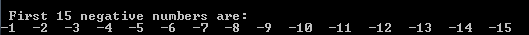Increment / Decrement factor need not be always incremented or decremented by 1. It can be by any number of factor. Consider the program to display first 50 even numbers and odd numbers. Note in below program how initial value, condition and increment factor. Here intNum is declared and initialized within the for loop. Hence its scope is only till the existence of the for loop. Hence we need to re-declare and initialize it in the for loop for odd number. If we had declared in the main function at its beginning, then its scope will remain throughout the existence of the main function. Hence we will not have to re-declare them in any of the for loops.

```#include

void main() {
printf("\n First 50 even numbers are: \n");
for (int intNum =2; intNum<=100 ; intNum+=2) {
printf("%d  ", intNum);
}

printf("\n First 50 odd  numbers are: \n");
for (int intNum = 1; intNum<=100; intNum += 2) {
printf("%d  ", intNum);
}
}```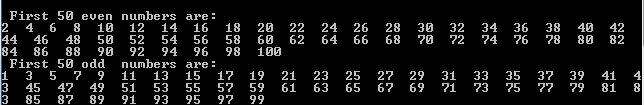We can even omit the increment/ decrement factors and use it inside the body of for loop. But a blank space needs to be kept after semicolon of condition.

```#include

void main() {
printf("\n First 50 even numbers are: \n");
for (int intNum =2; intNum<=100 ; ) {
printf("%d  ", intNum);
intNum += 2;
}
}```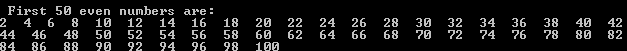From above programs we can conclude that we can have any kind of operators and conditions used in the ‘for‘loop. Only care should be taken not to deviate from the requirement.

## While and Do/While Loops

This is similar to for loops, but much simpler than it. While and do/while loops have similar functionality with small difference. The while loop checks for the condition/s and executes the set of statements within it until the condition is false. It does not require any initial values or increment factor. It always gets executed until the condition is false. In while loop, condition is checked at the beginning of the loop whereas in do/while loop condition is checked at the end of the loop. Hence in while loop, if the condition is false at the beginning itself, then it will not execute its body and comes out of the loop. But in do/while loop as the condition is checked at the end, it will execute body of it at least once.

### The general syntax for while and do/while loops are given below :

```while (condition/s){
Expression / statements;
}```
```do{
Expression / statements;
} while (condition/s);```

Like in for loops, these conditions can be anything. It can be a single condition with any operator or multiple conditions joined using logical operator.

Let us consider the same example of displaying first 15 natural numbers using both while and do while loops.

```#include

void main() {
int intNum = 0;

printf("\n Example of WHILE Loop\n");
printf("First 15 natural numbers are: \n");
while (intNum < 15){
printf("%d  ", intNum);
intNum++;
}

printf("\n Example of DO/WHILE Loop\n");
printf("First 15 natural numbers in descending order is: \n");
while (intNum >=0){
printf("%d  ", intNum--);
}
}```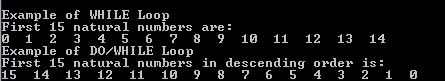In the example above, intNum is used to check for the condition and display the numbers. Initially it is declared and initialized. In while and do/while loops we cannot declare and initialize it like we did in for loops. This is because, these loops checks for the conditions and does not have any execution for the initial value. Hence if we declare or initialize the variable in while loop, it will check for the same condition repeatedly and the loop will never terminate.

When the while loop starts, it checks for the value in intNum with the condition, i.e.; 0

In the next lines it finds do/while loop. Here we have not initialized intNum and it has value 15. Since it is a do while loop, it does not checks for the condition first. Hence it enters the loop without any condition check. It prints the value of intNum which is 15. You will find the printf statement has postfix decrement operator on intNum – intNum –. Though we have decrement operator, printf statement prints 15 instead of 14. This is because of the priority of operator. Since it is a postfix operator, it first prints the value of intNum and then decrements the value of intNum. Hence intNum gets value 14 after printing it. Thus 15 is displayed at the first. Then comes the while condition. It checks if intNum >=0 → 14 >=0 → TRUE. Hence it repeats the loop and prints 14 and decrements the number. Again checks for condition and repeats the loop until it is false. i.e.; when intNum = -1, it checks -1 >=0 → FALSE. Hence comes out of the loop. Now intNum will have value -1. This is how both while and do/while loop works.

Here we have given the example of displaying the numbers with increment and decrement operators. It need not be increment / decrement by one. We can have any factor to increment or decrement. Even we can have multiplication or division or any other arithmetic expression, like in for loop.

Let us consider another example without any increment/decrement operator. Consider the program to enter the marks of the student from the keyboard till the user wants to enter the names.

```#include

void main() {
int intOption=1;
int intMark;

while (intOption ==1){
printf("\n Please enter the marks of a student:");
scanf("%d", &intMark);
printf("\n Do you want to continue(Yes - 1/ No - 0)?: \n");
scanf("%d", &intOption);
}
}```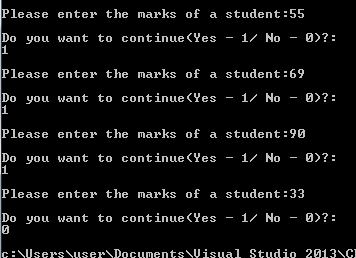Here the program checks for the user input value and the loop continues until they enter any other number other than 1, though it says 0 for No. this is because the condition in while loop is intOption == 1. This means when any other number is entered the condition returns FALSE and loop terminates.

Same can be re-written using do/while loop. Here initialization of intOption is not required as the condition is checked at the end. Hence it executes the loop at least once irrespective of the option being entered or whether it is initialized or not. Here you can note that we have entered option as 5 to terminate the loop even though it says 0 for No.

```#include

void main() {
int intOption;
int intMark;

do{
printf("\n Please enter the marks of a student:");
scanf("%d", &intMark);
printf("\n Do you want to continue(Yes - 1/ No - 0)?: \n");
scanf("%d", &intOption);
} while (intOption == 1);
}```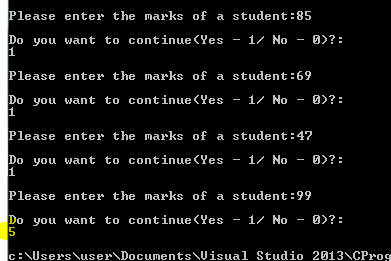Translate »